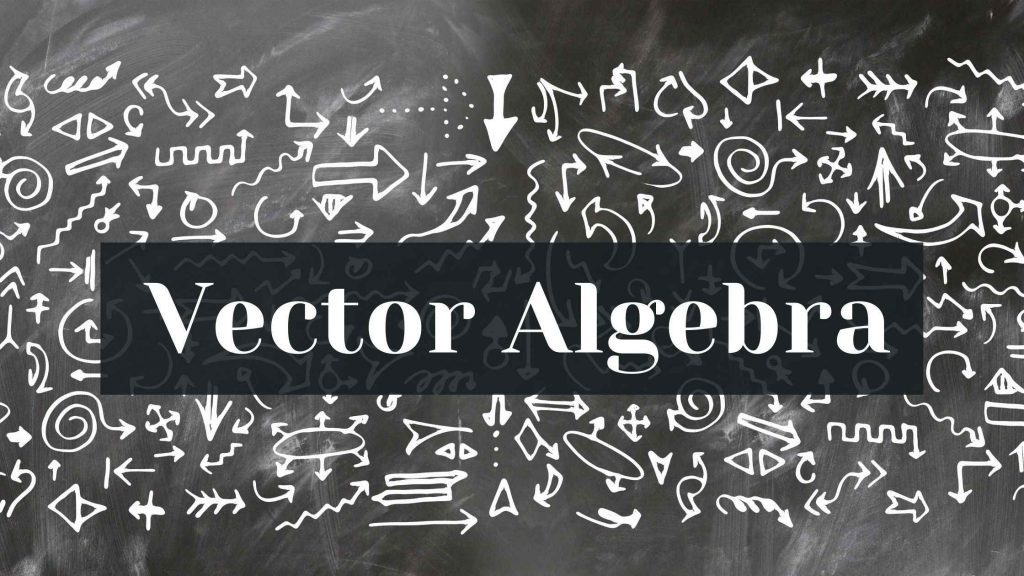Maths
Study Material

# Vector Algebra Class 12

Vector algebra deals with vectors, things that have both magnitude and direction. Read here more about the basic concepts of vectors.

4 minutes longHesap Oluştur

Got stuck on homework? Get your step-by-step solutions from real tutors in minutes! 24/7. Unlimited.

Vector algebra deals with vectors, things that have both magnitude and direction. Like forces, that have directions and magnitudes. Vector algebra may be a must in such situations of operations on forces because you’ll not combine them like just numbers (that have only magnitude and no direction). Here we will go through the introduction of vectors.(vector algebra class 12)

## What is a Vector?

Vector is defined as the quantity consisting of both magnitude and direction. It represents the direction of objects from one position to another. We can say that the starting point is the tail and the ending point is the head.
The distance between initial and terminal points of a vector is called the magnitude of the vectors.

## Examples of Vectors

Examples of the vector are Velocity, Acceleration, Force, Increase-Decrease in Temperature etc. All these quantities have directions and magnitude both. Therefore, it’s necessary to calculate them in their vector form.
Also, speed is quantity that has magnitude but no direction.

## Types Of Vectors

• Zero Vector : We can say, a vector whose magnitude is zero.
• Unit Vector : We can say, a vector with magnitude of one unit.
• Position Vector : We can say, a vector that denotes the position of a point with respect to its origin.
• Co Initial Vector : We can say, two or more vectors with the same starting point.
• Co-planar Vector : Vectors in the same plane.
• Co-linear Vector : Vectors lying on the same straight line.
• Equal Vector : Two or more vector with the same magnitude and direction.
• Displacement Vector : A vector indicating displacement of an object from one point to another.
• Negative of a Vector : Negative of any vector is another vector with the same magnitude but opposite direction.

## Unit Vector

A unit vector has a length/magnitude equal to one, which is basically used to show the direction of any vector. We say, a unit vector is equal to the ratio of a vector and its magnitude. Represented by a cap or hat (^).

• If n is vector of arbitrary length and its magnitude is |n|, then the unit vector is given by:
• n̂ = n/∣n∣

Given two vectors a and b, we form their sum a+b, as follows. We translate the vector b until its tail coincides with the head of a. And then, the directed line segment from the tail of a to the head of b is the vector a+b.
Addition of vector satisfies two important properties.

• The commutative law, which states the order of addition doesn’t matter:
• a+b=b+a.
• The associative law, which states that the sum of three vectors does not depend on which pair of vectors is added first:
• (a+b)+c=a+(b+c).

## Vector subtraction

We define subtraction as addition with the opposite of a vector, as shown: b−a=b+(−a).

## Scalar Multiplication

Given a vector a and a real number (scalar) λ, we can form the vector λa as follows. If λ is positive, then λa is the vector whose direction is the same as the direction of a and whose length is λ times the length of a. In this case, multiplication by λ simply stretches (if λ>1) or compresses (if 0<λ<1) the vector a.

## Vector Multiplication

• Multiplication of any two vectors is performed by finding their
• Cross product and Dot product
• Mathematically Cross Product of Two Vectors a and b is :
• a×b =|a||b| sin θ n̂
• where, |a| is the magnitude of vector ‘a’
• |b| is the magnitude of vector ‘b’
• θ is the angle of separation of two vectors ‘a’ and ‘b’
• n̂ is the unit vector representing the direction of multiplication of vectors
• Mathematically Dot Product of Two Vectors a and b is :
• a . b =|a||b| cos θ
• where, |a| is the magnitude of vector ‘a’
• |b| is the magnitude of vector ‘b’
• θ is the angle of separation of two vectors ‘a’ and ‘b’
• Cross product of two vectors is a vector quantity. It has both magnitude and direction whereas, the dot product of two vectors has only magnitude and no direction. So, it is a scalar quantity. (vector algebra class 12)